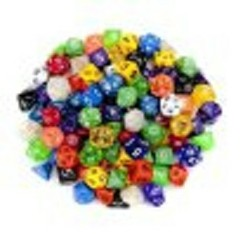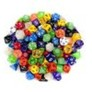# Probability for Homeschoolers and Others Studying Math

## Probability does not have to be difficult. This is an easily understood approach.

Probability for Homeschoolers and Others Studying Math

Are you teaching a homeschooler probability, helping a student in school with probability, or just interested in learning for yourself? Well, probability does not have to be difficult. In fact, it can be one of the easiest of topics in mathematics to explain and to understand. If you can work with fractions, or have a calculator that can, you can handle probability. Just remember a few things, and the problems will be easy. How easy? You will become so confident you will be able to teach your homeschooler, or other student.

Intro Image, and all other images: Allowed by Amazon affiliate program

Simply put, the probability of something occurring is the number of favorable occurrences, called events in formal mathematics, divided by the total number of possible occurrences, provided all occurrences are equally likely.  It is that equally likely part you must pay attention to.

A simple case is to roll a numbered, six-sided die, and ask the probability of a side coming up.  Well, the probability of the side numbered one coming up is one-sixth, there is only one favorable side, divided by six, there are six sides.  If you ask the probability any other number will come up the answer will still be one divided by six, since no matter which number you ask about it is only on one side.  You would write 1/6.

Now let’s ask what is he probability an even number comes up when the same die is rolled.  There are now three favorable outcomes, the two, four, or six coming up, and still six total possible outcomes, so the answer is three divided by six.  You would write 3/6, or 1/2.

So, the first thing you must do is count.  Count the favorable outcomes and count the total outcomes.

Remember, the probability cannot be less than zero, nor greater than one.  If something cannot happen, such as a seven come up with the same numbered die being rolled, there are zero favorable outcomes.  Zero divided by six is still zero.  On the other hand, the probability of a number from one through six, inclusive, coming up is six divided by six, or one.  At least one of the numbers must come up.  If something must happen, the probability is one.

Now this does not look difficult, does it?

### Probability when Outcomes Do Not Look Equally Likely

##### Making Outcomes Equally Likely

Suppose you have a bag containing six marbles.  Three of the marbles are red, two are blue, and one is white.  If I ask you what is the probability of reaching into the bag and picking a red marble, you just know it is not one divided by three.   But, there are three colors possible.  Since they are not equally likely, adjust the problem to have six, equally likely outcomes.  Now there are three red marbles, or three favorable outcomes, and six total marbles.  The probability of picking a red marble is 3/6, or 1/2.

It is important to remember that before you start, make certain the outcomes are equally likely, or adjust them to be so before going on.

### Probability Resources

##### Spinners, Dice, and Disks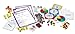Learning Resources Deluxe Probability KitView on Amazon

### Conditional Probability

##### What if I Know Something?

Sometimes you may know something that will change the probability.  Suppose you take a deck of cards, a standard fifty-two card deck.  If I ask you what is the probability there is a king as the top card, there are four kings, which constitute the favorable outcomes, and fifty-two possible outcomes.  The answer would be 4/52, or 1/13.

Now let’s change the problem slightly.  Before placing the deck of cards on the table, I show you the bottom card.  Well, now you know something.  The bottom card cannot be the top card, so there are only fifty-one possible outcomes.  If the bottom card is a king, there are three possible kings to be the top card, so the probability is 3/51.  If the bottom card is not a king, any of the four kings might be the top card, so the probability is 4/51.

See how things change if you know something.

### Using Math Manipulatives for Problem Solving Series: Investigating Probability--Using Logical Reasoning

##### DVDUsing Math Manipulatives for Problem Solving Series: Investigating ...View on Amazon

### Odds

##### Not Exactly Probability

The odds in favor of something happening is calculated by the favorable outcomes divided by the unfavorable outcomes.

What are the odds of a king being on the top of a fifty-two card deck?  There are four favorable outcomes, and forty-eight unfavorable outcomes.  So, the odds of having a king on top of a deck of cards is 4/48, or 1/12.

Notice the difference.

### Polyhedral Dice

##### Large Pack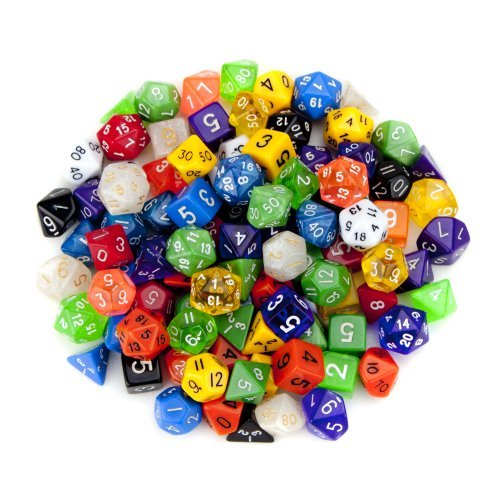Wiz Dice Random Polyhedral Dice in Multiple Colors (100 + Pack) Bun...View on Amazon

### Polyhedral Dice

Why would you need this many polyhedral dice?  well, if I roll a single die once, one number comes up.  It gives me no idea f what would be the frequency of having that number come up.  To roll a single die hundreds of times takes too long, so if each time a handful of identical dice are rolled it will not take so long.  It is an easy and fast way of repeating an experiment many times.

Color is not an important factor here, the number of sides is.

The key here is a large number of times.

Notice I did not say flipping a coin.  This is because the weight is not distributed equally, so one side tends to land down a slightly greater number of times than the other.    After all, the government is not making coins for probability experiments, so they really don’t care.

### Complement

Since something must either be favorable or unfavorable, the probability of something occurring plus the probability of that same something not occurring must be one.  This can be rewritten to say the probability of the complement of something occurring, that is something not occurring, is one minus the probability the having the same something occur.

This article contains links to affiliate programs from and Adsense advertising.  These must use cookies to allow for proper crediting. As an Amazon Associate I earn from qualifying purchases.

Updated: 07/30/2018, blackspanielgallery
Thank you! Would you like to post a comment now?
3

Only logged-in users are allowed to comment. Login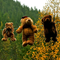sheilamarie on 06/19/2015

It's always good to have an article explaining math concepts in a simple way. Thanks.

#### You might also like

Helping Children with Arithmetic: The Early Grades

Online classes were not good for education, especially arithmetic classes. N...

Learning Math Is Possible

Learning math is possible, but there are a few things that you should know be...

Disclosure: This page generates income for authors based on affiliate relationships with our partners, including Amazon, Google and others.